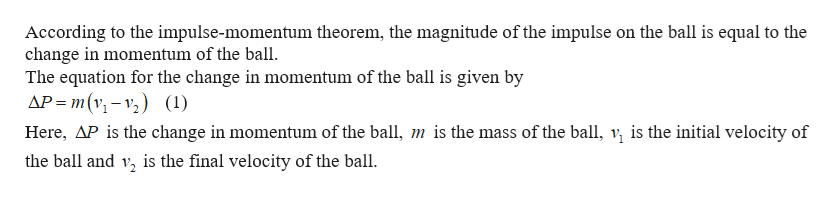# A 0.8 kg ball is traveling at 4.0 m/s and strikes a wall. The ball bounces off the wall with a velocity of 4.0 m/s in the opposite direction. If the contact with the wall by the ball lasts for 0.13 seconds, then the magnitude of the impulse on the ball is

Question

A 0.8 kg ball is traveling at 4.0 m/s and strikes a wall. The ball bounces off the wall with a velocity of 4.0 m/s in the opposite direction. If the contact with the wall by the ball lasts for 0.13 seconds, then the magnitude of the impulse on the ball is

check_circleExpert Solution
Step 1help_outlineImage TranscriptioncloseAccording to the impulse-momentum theorem, the magnitude of the impulse on the ball is equal to the change in momentum of the ball The equation for the change in momentum of the ball is given by AP m(v (1) Here, AP is the change in momentum of the ball, m is the mass of the ball, v is the initial velocity of the ball and v, is the final velocity of the ball fullscreen

### Want to see the full answer?

See Solution

#### Want to see this answer and more?

Solutions are written by subject experts who are available 24/7. Questions are typically answered within 1 hour*

See Solution
*Response times may vary by subject and question
Tagged in

### Kinematics